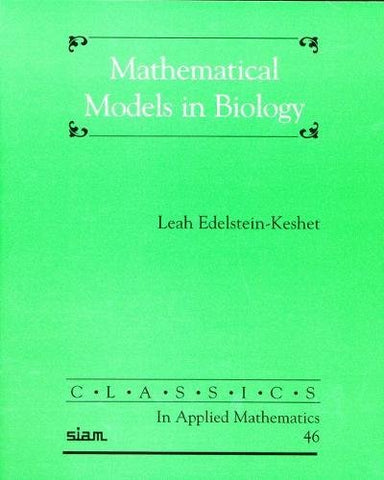# Mathematical Models in Biology (Classics in Applied Mathematics)

• ISBN-13: 9780898715545
• \$41.75

Only 3 left!

Mathematical Models in Biology is an introductory book for readers interested in biological applications of mathematics and modeling in biology. Connections are made between diverse biological examples linked by common mathematical themes, exploring a variety of discrete and continuous ordinary and partial differential equation models. Although great advances have taken place in many of the topics covered, the simple lessons contained in Mathematical Models in Biology are still important and informative. Shortly after the first publication of Mathematical Models in Biology, the genomics revolution turned Mathematical Biology into a prominent area of interdisciplinary research. In this new millennium, biologists have discovered that mathematics is not only useful, but indispensable! As a result, there has been much resurgent interest in, and a huge expansion of, the fields collectively called mathematical biology. This book serves as a basic introduction to concepts in deterministic biological modeling.